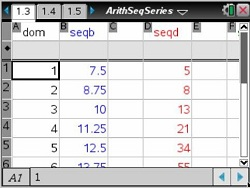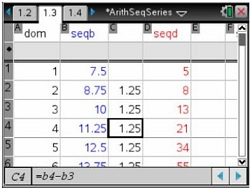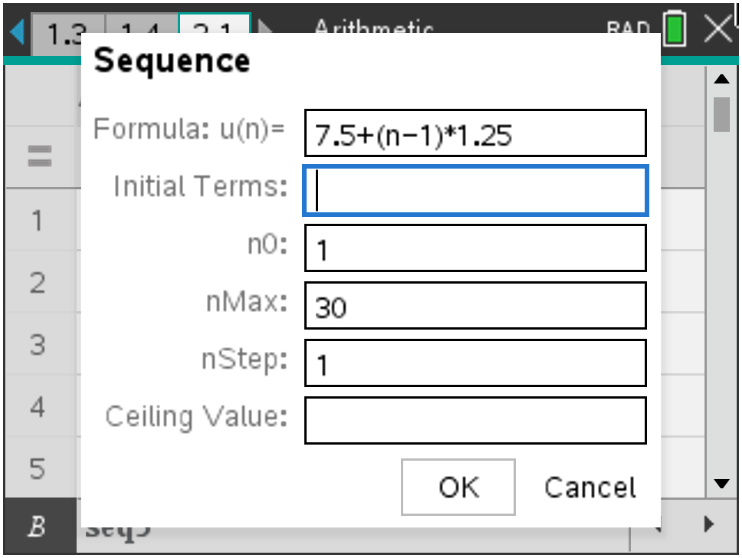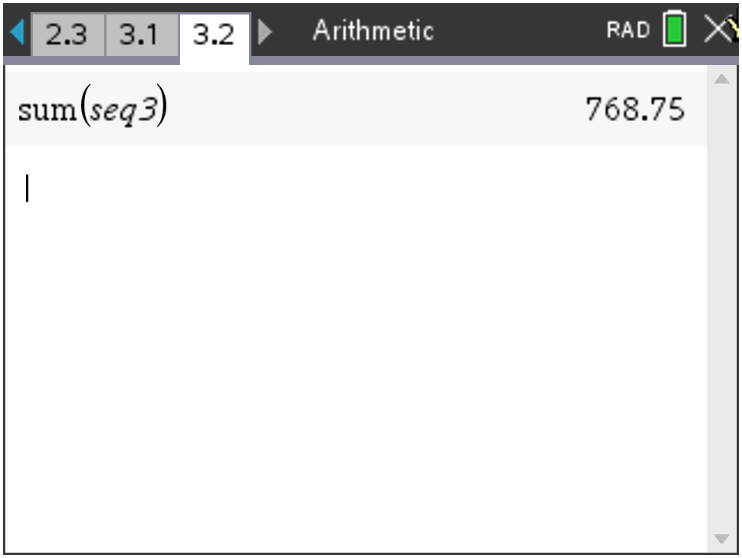### Mathematics lessons for IB® Diploma Programme

Applications and Interpretation | Numbers and Algebra## Arithmetic Sequences and Series

### Activity Overview

Use formulas to find the differences of the consecutive terms, plot a scatter plot of each sequence, and determine the shape of sequences with common differences. Then use a spreadsheet to display the first n terms and find the sum.

## Key Steps## Step 1

Students will use formulas to find the differences of the consecutive terms of a sequence.## Step 2

Then, students will plot a scatter plot of each sequence and determine that sequences with common differences, also called arithmetic sequences, have scatter plots that form a straight line.## Step 3

Students will find the sum of the first n terms of a sequence using a formula and using sigma notation.